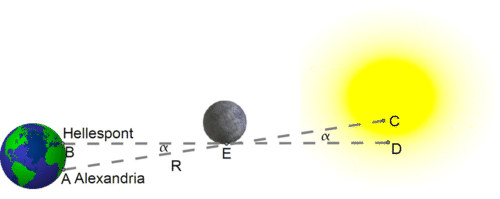20.1: The Eclipse

•• Contributed by CK12
• CK12Figure $$\PageIndex{1}$$: Moon blocking the Sun during a solar eclipse (not to scale)

In a total eclipse of the Sun, the Moon just barely covers the Sun. The Sun itself is so distant that when viewed from anywhere on Earth, it covers practically the same patch of the sky, with a width of about 0.5 degrees. Hipparchus concentrated on point E at the edge of the Moon (drawing), which during totality, when viewed from the Hellespont (point B) just overlapped point D on the edge of the Sun.

Viewed from Alexandria (point A), at that same moment, the point E only overlapped point C on the Sun, about 1/5 solar diameter short of the edge --- which was why the eclipse there was not total. One-fifth of the Sun's diameter covers about 0.1 degrees in the sky, so the small angle $$\alpha$$ (alpha, Greek A) between the two directions measured about 0.1 degrees. That angle is the parallax (See the chapter “Parallax") of the edge of the Moon, viewed from the above two locations.

It is unlikely that Hipparchus knew the distance AB, but he probably knew the latitudes of the Hellespont and Alexandria. The local latitude can be shown to be equal to the elevation of the celestial pole above the horizon and today can be readily deduced by observing the height of Polaris, the pole star above the horizon. In the time of Hipparchus the pole of the heavens wasn't near Polaris (because of the precession of the equinoxes), but Hipparchus, who had mapped the positions of about 850 stars, must have known its position quite well.

The latitude of the Hellespont (from a modern atlas) is about 4020′ (40 degrees and 20 minutes, 60 minutes per degree), while that of Alexandria is about 3120′, a difference of 9 degrees. We will also assume Alexandria is exactly due south. Furthermore, if $$r$$ is the radius of the Earth, then the circumference of the Earth is $$2\pi r$$. Since the circumference also spans 360 degrees, we get $AB\,=\,\frac{2\pi r}{360}\times 9$